# Chapter 5: Periodic Classification of Elements

Course Content
Science (NCERT Solutions)
0/16
Science (MCQ)
0/16
Science (Video Lessons in Assamese)
0/7
Science (Test Yourself)
0/1
Maths (Video Lessons in Assamese)
Maths (MCQ)
0/8
Maths (Test Yourself)
0/3
Maths (1 mark question)
0/4
Class 10 : A Complete Package

## Chapter 5: Periodic Classification of Elements

Q1) Which of the following forms the basis of the modern periodic table?

a) Atomic mass

b) Atomic number

c) Number of nucleons

d) All of the above

Q2) Which of the following is the most reactive element of group 17?

a) Oxygen

b) Sodium

c) Fluorine

d) Magnesium

Q3) Which of the following is the correct order of the atomic radii of the elements oxygen, fluorine and nitrogen?

a) O < F < N

b) N < F < O

c) O < N < F

d) F < O < N

Q4) Element X forms a chloride with the formula XCl2, which is solid with a high melting point. X would most likely be in the same group of the Periodic Table as:

a) Na

b) Mg

c) Al

d) Si

Q5) What happens to the electropositive character of elements on moving from left to right in a periodic table?

a) Increase

b) Decreases

c) First increases, then decreases

d) First decreases, then increases

Q6) What is the other name for group 18th elements?

a) Noble gases

b) Alkali metals

c) Alkali earth metals

d) Halogens

Q7) Which group elements are called transition metals?

a) Group number 1 to 2

b) Group number 13 to 18

c) Group number 3 to 12

d) Group number 1 to 8

Q8) The electronic configuration of an element M is 2, 8, 4. In the modern periodic table, the element M is placed in

a) 4th group

b) 2nd group

c) 14th group

d) 18th group

Q9) Which of the following elements has 2 shells, and both are completely filled?

a) Helium

b) Neon

c) Calcium

d) Boron

Q10) Which of the following option describes the achievements of Mendeleev’s Periodic Table?

(a) Prediction of noble gases

(b) It eliminated the blank spaces left in the table

(c) Predicting that the elements can be arranged based on their properties

(d) An element in a trend has an average atomic mass of the elements above and below it

Q11) The following image shows an element with its atomic number and mass number.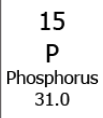Which option arranges the element in the periodic table?

(a) Group – 1; period – 1

(b) Group – 5; period – 3

(c) Group – 10; period – 1

(d) Group – 15; period – 3

Q12) A student learns that the atomic size depends on the atomic radius of the elements. How does the atomic radius of elements in the third-period change as one goes from sodium to argon?

(a) Atomic radius increases from sodium to argon

(b) Atomic radius decreases from sodium to argon

Q13) Boron is a non-metal and is placed under group 13 and period 2. How can boron form bonds with other elements?

(a) By sharing 5 electrons

(b) By sharing 3 electrons

(c) By sharing 2 electrons

(d) By sharing 1 electron

Q14) Electronegativity is defined as the ability of an element to form bonds by gaining electrons. How does the electronegativity of elements vary across the periods?

(a) It increases as the number of shells increases

(b) It decreases as the number of shells decreases

(c) It increases as more electrons are added to the same shell

(d) It decreases as more electrons are added to the same shell

Q15) What is the order of the metallic character down the group?

(a) It decreases as new shells are added to the element

(b) It increases as electrons move away from the nucleus

(c) It increases as new atoms are added to the same shell

(d) It decreases as the effective nuclear charge on the electron increases

Q16) An element X has the atomic number 9. In which period and group can it be placed in the modern periodic table?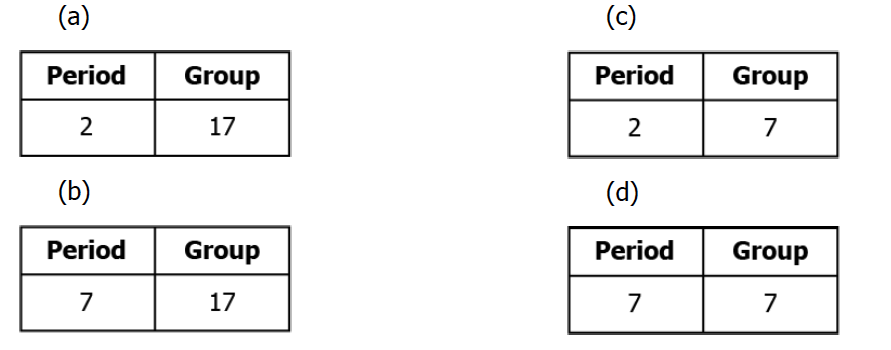Q17) A student studying the modern periodic table arranges some elements in different groups, as shown below: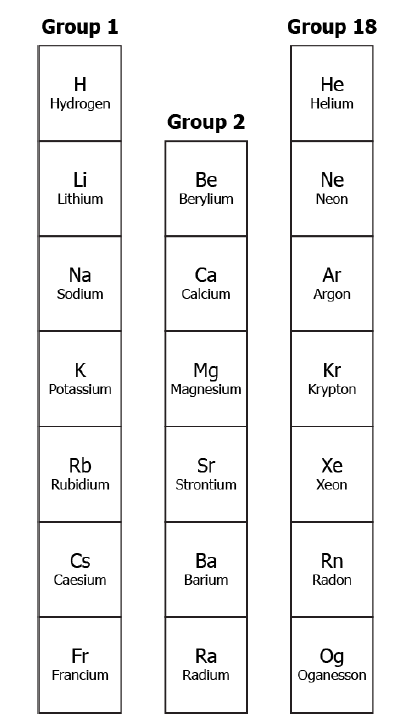Which group supports the guidelines of the modern periodic table?

(a) Group 1

(b) Group 18

(c) Groups 1 and 2

(d) Groups 1 and 18

Q18) A student studies Mendeleev’s periodic table and lists some statements.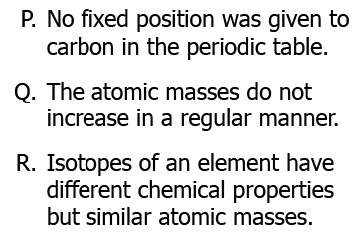Which option lists the limitations of Mendeleev’s periodic table?

(a) Only P

(b) Only R

(c) Both P and Q

(d) Both Q and R

Q19) Which option arranges the elements of period four in the correct groups?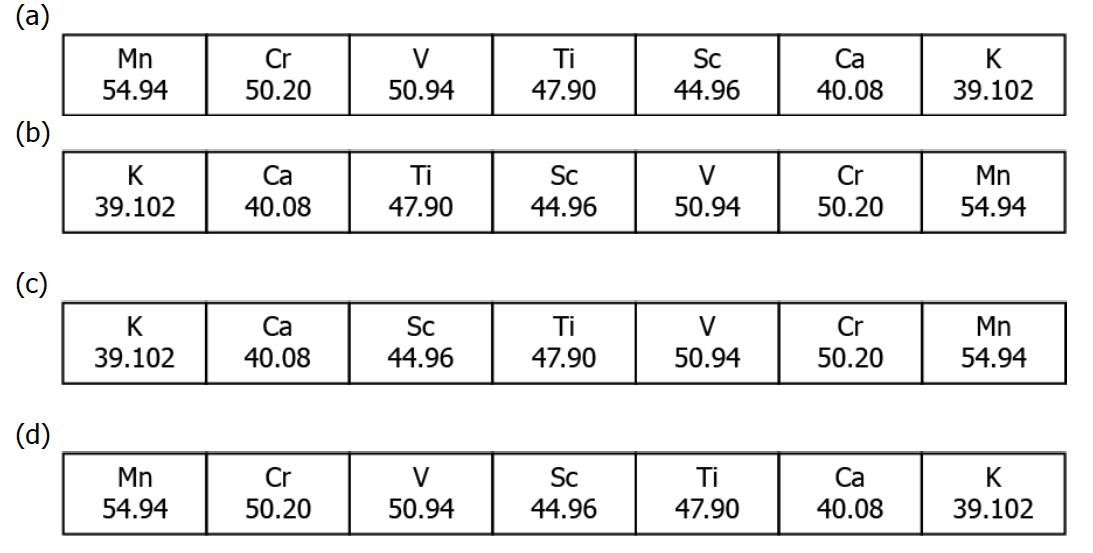Q20) What is the trend of valency along the periods in the modern periodic table?

(a) It increases from left to right

(b) It decreases from right to left

(c) It increases and then decreases

(d) It decreases and then increases